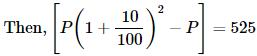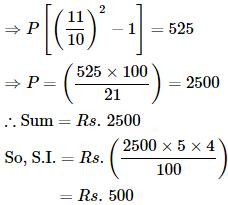Courses

# Test: Compound Interest- 2

## 10 Questions MCQ Test IBPS PO Mains - Study Material, Online Tests, Previous Year | Test: Compound Interest- 2

Description
This mock test of Test: Compound Interest- 2 for Quant helps you for every Quant entrance exam. This contains 10 Multiple Choice Questions for Quant Test: Compound Interest- 2 (mcq) to study with solutions a complete question bank. The solved questions answers in this Test: Compound Interest- 2 quiz give you a good mix of easy questions and tough questions. Quant students definitely take this Test: Compound Interest- 2 exercise for a better result in the exam. You can find other Test: Compound Interest- 2 extra questions, long questions & short questions for Quant on EduRev as well by searching above.
QUESTION: 1

Solution:
QUESTION: 2

### The compound interest on a certain sum for 2 years at 10% per annum is Rs. 525. The simple interest on the same sum for double the time at half the rate percent per annum is:

Solution:

Let the sum be Rs. PQUESTION: 3

### The Simple interest on a certain sum for 2 years at 20% per annum is Rs. 80. The corresponding compound interest is

Solution:
QUESTION: 4
Arun invested an amount of Rs. 20000 in a fixed deposit scheme for 2 years at compound interest rate 4 p.c.p.a. How much amount will Arun get on maturity of the fixed deposit?
Solution:
QUESTION: 5

The compound interest on Rs. 20,480 at 6 1/4 % per annum for 2 years 73 days, is:

Solution:
QUESTION: 6

A sum put out at 4% compound interest payable half-yearly amounts to Rs. 13265.10 in 1 1/2 years. The sum is:

Solution:
QUESTION: 7
The least number of complete years in which a sum of money put out at 20% compound interest will be more than doubled is
Solution:
QUESTION: 8
Simple interest on a certain sum of money for 4 years at 5% per annum is half the compound interest on Rs. 3000 for 2 years at 10% per annum. The sum placed on simple interest is:
Solution:
QUESTION: 9
The difference between simple interest and compound on Rs. 2400 for one year at 10% per annum reckoned half-yearly is:
Solution:
QUESTION: 10
A sum amounts to Rs. 882 in 2 years at 5% compound interest. The sum is
Solution: